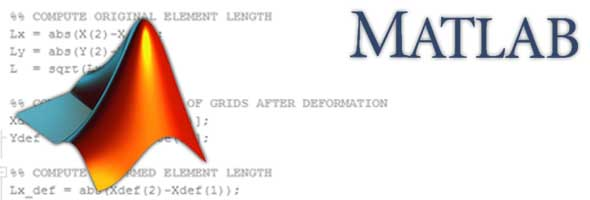# What is MATLAB?

MATLAB (short for MATrix LABoratory) is a high-level computer programming language used across a variety of engineering industries and academics. It is developed by MathWorks. Among other things, it's useful for:

• Mathematical computations
• Modeling and simulations
• Data analysis and post-processing
• Visualization and graphics
• Algorithm development### Why do Engineers like MATLAB?

Like it or not, computer programming is an integral part of many engineering jobs in the modern day. It seems some people love programming and others simply tolerate programming. Regardless of how you feel, MATLAB has a number of features that make things easier when compared to many other programming languages for many engineering applications.

Scientific - MATLAB was developed with scientific computing in mind. This means that the mathematical operations engineers and scientists use most are built in and easily implemented!

Scripted - MATLAB is a scripted programming language. In contrast with other compiled languages, like Fortran or C, instructions you write in MATLAB are ready to perform tasks as soon as they hit the screen! You can also easily transfer programs to any computer with a MATLAB installation.

Toolboxes - MATLAB toolboxes are collections of functions that are specific to a particular task or field. For example, the Aerospace toolbox includes programs for determining atmospheric data, performing lift calculations, and numerous other tasks that would help an aerospace engineer design or analyze an airplane.

Simple Syntax - the syntax of a programming language is its grammar and vocabulary. This set of rules that govern the structure of a program in MATLAB is straightforward, which helps decrease development time!

Now that you know what MATLAB is, lets now head on to the next part of this tutorial and learn about the parts of the MATLAB environment: Getting to Know the MATLAB Environment.

icon-long-arrow-left Last icon-cog Next icon-long-arrow-right